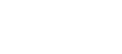# Bernoullis Theorem

Bernoulli's theorem is a method of expressing the law of energy conservation to the flow of fluids.Bernoulli's principle states that, in the flow of fluid (a liquid or gas), an increase in velocity occurs simultaneously with a decrease in pressure. That statement is a simplification of Bernoulli's equation (below) which plots the situation at any point on a streamline of the fluid flow and applies the law of conservation of energy to flow. Put another way, the total energy of the flow at any point along a horizontal pipe is equal to the sum of the pressure head, the velocity head and the elevation in the absence of friction. This is a principle of considerable importance to those concerned with the flow in sprinkler pipework.When

z = Potential head or elevation
p = Pressure
v = Velocity
g = Acceleration of gravity
d = Density of fluid